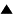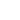# Statement of infiniteness

[Node to be completed]

The statement that a certain process is infinite is not a prediction. But it is a generator of predictions. Our definition of a prediction T! includes a procedure, let it be denoted as P(t_i), which is applied to the states t_i, i= 1,2,... etc. of the test T and determines, in a finite time, whether each given state is Success, Failure, or neither of the two, which we shall denote as Not-end. Therefore, the statement that the state t_i is Not-end is a prediction, namely, the success of the procedure that runs P(t_i) and compares the result with Not-end. Now we can define what is the meaning of the statement that T is infinite: it is a generator which produces the following row of predictions:

t_1 is Not-end

t_2 is Not-end

t_3 is Not-end

...

etc., infinitely

 HomeMetasystem Transition TheoryEpistemologyKnowledgeTruth Up Prev.Next Down